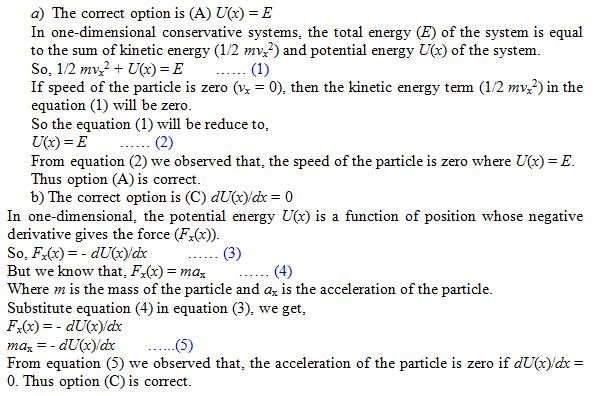×#### Thank you for registering.

One of our academic counsellors will contact you within 1 working day.

Click to Chat

1800-1023-196

+91-120-4616500

CART 0

• 0

MY CART (5)

Use Coupon: CART20 and get 20% off on all online Study Material

ITEM
DETAILS
MRP
DISCOUNT
FINAL PRICE
Total Price: Rs.

There are no items in this cart.
Continue Shopping
```
A particle with total energy E moves in one dimension in a region where the potential energy is U (x). (a) The speed of the particle is zero where (A) U(x) = E. (B) U(x) = 0. (C) dU(x)ldx = 0. (D) d 2 U(x)ldx 2 = 0. (b) The acceleration of the particle is zero where (A) U(x) = E. (B) U(x) = 0. (C) dU(x)ldx = 0. (D) d 2 U(x)ldx 2 = 0.
A particle with total energy E moves in one dimension in a region where the potential energy is U (x).(a) The speed of the particle is zero where(A) U(x) = E.                     (B) U(x) = 0.(C) dU(x)ldx = 0.(D) d2U(x)ldx2 = 0.(b) The acceleration of the particle is zero where(A) U(x) = E.                     (B) U(x) = 0.(C) dU(x)ldx = 0.                                           (D) d2U(x)ldx2 = 0.

```
5 years ago

``````
5 years ago
Think You Can Provide A Better Answer ?

## Other Related Questions on Mechanics

View all Questions »### Course Features

• 101 Video Lectures
• Revision Notes
• Previous Year Papers
• Mind Map
• Study Planner
• NCERT Solutions
• Discussion Forum
• Test paper with Video Solution### Course Features

• 110 Video Lectures
• Revision Notes
• Test paper with Video Solution
• Mind Map
• Study Planner
• NCERT Solutions
• Discussion Forum
• Previous Year Exam Questions